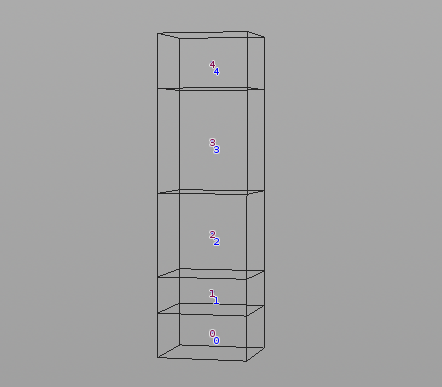## Recommended Posts

Hello,

Is anyone able to point out where I am going wrong here? Ideally I would like the boxes of different scales to stack up one on top of another.

In another package, using python on a series of boxes I get the desired stacking effect. When I try to convert the python to vex it's not working as I'd hoped. I suspect it's a really small oversight on my behalf but it would be really helpful for me to understand where I'm doing wrong.

My Python code

```# Example Python code

def main():
list = op.GetObjects()
new = Vector(0)

for object in list:
pos = object.GetPos()
scale = object.GetScale()

new.y = new.y + scale.y + pos.y

#Set new position to new
object.SetPos(new)

#Update new
new.y = new.y + scale.y - pos.y```

My Vex code run in Detail mode

```vector new = 0;
for (int i = 0; i < (@numpt); i++){
vector pos = point(0, "P", i);
vector scale = point(0, "scale", i);

new.y = new.y + scale.y + pos.y;

setpointattrib(0, "P", i, new);

new.y = new.y + scale.y - pos.y;
}```

Thank you

Edited by sessionbeer

##### Share on other sites
```//point wrangle
@P.y += v@scale.y;```

not sure if this is a small example of a larger problem you're trying to solve. sorry if this is obvious and not what you're looking for

if you still want to use a detail wrangle

```for (int i = 0; i < (@numpt); i++)
{
vector pos = point(0, "P", i);
vector scale = point(0, "scale", i);
pos.y += scale.y;
setpointattrib(0, "P", i,  pos);
}```

Edited by dchow1992

##### Share on other sites

Thanks for taking a look @dchow1992

With that code the boxes won't stack one on top of another, they simply move up the size of their scale.y

I'm looking to get boxes of different scales to sit one on top of another.

##### Share on other sites

Hey Sessionbeer,

Slightly different setup but it works on a detail wrangle:

```//get start point
vector new = point(0,"P",0);

for (int i = 0; i < @numpt; i++){

if(i == 0) // skip first point
continue;

vector scale = point(0, "scale", i);
vector prev_scale = point(0, "scale", i-1);

//get the height fron current and prev_scale combined
new.y = new.y + ((scale.y + prev_scale.y)*0.5);

setpointattrib(0, "P", i, new);
}```•1

##### Share on other sites

Thanks @Zybrand That worked out great!•1

##### Share on other sites

Just a note that when you run VEX in Detail mode you lose the multi-processor benefit of VEX. So you may not see a big performance benefit converting from Python to VEX in this case.

## Create an account

Register a new account